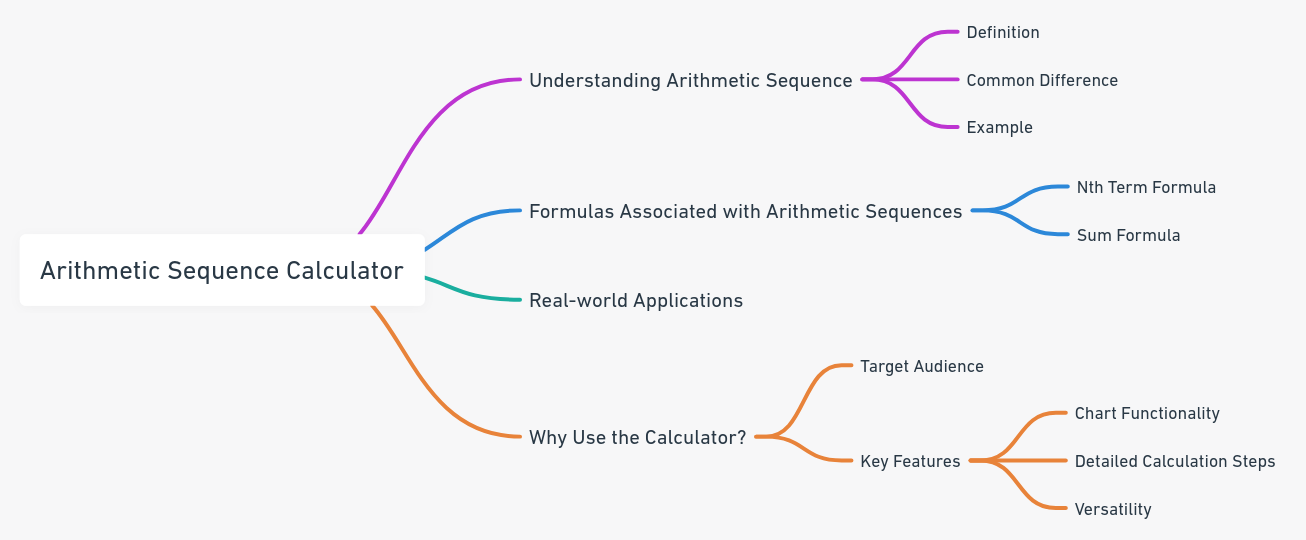Even The mini Tools Can Empower People to Do Great Things.

# Arithmetic Sequence Calculator: Chart Your Way to Sequence Mastery with Full Calculation Steps.

Discover the precision of our Arithmetic Sequence Calculator. Effortlessly find the nth term and sum of sequences, visualized through detailed charts.

Arithmetic Sequence Calculator (High Precision)
 First term of the sequence (a1): Common difference (d): Enter n: Precision: 10 20 30 40 50 60 70 80 90 100 200 300 400 500 600 700 800 900 1000

Embed Arithmetic Sequence Calculator (High Precision) Widget

## About Arithmetic Sequence Calculator (High Precision)Harness the power of our Arithmetic Sequence Calculator to determine both the nth term and the cumulative sum of the initial n terms in an arithmetic sequence. With a step-by-step approach and intuitive chart functionality, mastering sequences has never been easier.

## Understanding Arithmetic Sequence

An arithmetic sequence, often termed an arithmetic progression, is a foundational concept in mathematics that represents a series of numbers. The unique characteristic of this sequence is the constant difference between its consecutive terms. This constant difference, known as the "common difference," remains the same throughout the sequence, giving it a structured and predictable pattern.

For instance, consider the sequence 2, 4, 6, 8, 10. Here, the common difference is 2, as each term increases by 2 from its predecessor.

### Formulas Associated with Arithmetic Sequences:

If the initial term of an arithmetic sequence is a1 and the common difference of successive members is d, then the nth term of the sequence is given by:

an = a1 + (n - 1)d

The sum of the first n terms Sn of an arithmetic sequence is calculated by the following formula:

Sn = n(a1 + an)/2 = n[2a1 + (n - 1)d]/2

Arithmetic sequences are prevalent in various real-world scenarios, from financial calculations to natural phenomena. Understanding the sequence and its formulas can provide valuable insights into patterns, predictions, and problem-solving in diverse fields.

## Why Use the Arithmetic Sequence Calculator?

Whether you're a student grappling with homework, a teacher crafting lesson plans, or just a curious mind, the Arithmetic Sequence Calculator is your ultimate ally. Not only does it provide accurate results, but its chart function visually represents the sequence, aiding comprehension. Moreover, the full calculation steps ensure you're never lost in the process.

### Key Features:

1. Chart Functionality: Visualize your arithmetic sequence, understanding patterns and trends at a glance.
2. Detailed Calculation Steps: From pinpointing the common difference to computing the nth term using the formula an=a1+(n-1)d, our calculator provides a comprehensive breakdown.
3. Versatility: Whether you're solving a sequence with the arithmetic sequence solver or calculating a series sum with the arithmetic series calculator, this tool caters to all your needs.